• 一维中值滤波函数，调用函数，输入滤波窗口值和初始一维滤波向量，输出滤波后的向量
• MATLAB 自适应中值滤波函数adpmedian参考 冈萨雷斯 《数字图像处理》MATLAB版function f = adpmedian(g,Smax)if (Smax<=1)||(Smax/2==round(Smax/2))||(Smax~=round(Smax))error('Smax must be an odd integer &...
MATLAB  自适应中值滤波函数adpmedian参考 冈萨雷斯 《数字图像处理》MATLAB版function f = adpmedian(g,Smax)if (Smax<=1)||(Smax/2==round(Smax/2))||(Smax~=round(Smax))error('Smax must be an odd integer >1.')endf=g;f(:)=0;alreadyProcessed=false(size(g));for k=3:2:Smaxzmin=ordfilt2(g,1,ones(k,k),'symmetric');zmax=ordfilt2(g,k*k,ones(k,k),'symmetric');zmed=medfilt2(g,[k k],'symmetric');processUsingLevelB=(zmed>zmin)&(zmax>zmed)&...~alreadyProcessed;zB=(g>zmin)&(zmax>g);outputZxy=processUsingLevelB & zB;outputZmed=processUsingLevelB&~zB;f(outputZxy)=g(outputZxy);f(outputZmed)=zmed(outputZmed);alreadyProcessed=alreadyProcessed | processUsingLevelB;if all(alreadyProcessed(:))break;endendf(~alreadyProcessed)=zmed(~alreadyProcessed);end
展开全文• >> median(A,1) //列的中值 ans =         2        7        5 >> median(A,2)//行的中值 ans =         4         3         7


median的作用：求矩阵均值。

median(M),每一列返回一个值,为M该列的从大到小排列的中间值.

median(M,dim),dim为1，2。其中1表示按每列返回一个值,为该列从大到小排列的中间值,,

2表示按每行返回一个值,为该行从大到小排列的中间值.

注意:如果行或列的个数为偶数,返回中间两个值的平均值。举例如下：

A=[1 4 5;2 8 3;9 7 6];
>> median(A)

ans =

2     7     5

>> median(A,1)   //列的中值

ans =

2     7     5

>> median(A,2)//行的中值

ans =

4
3
7


展开全文• 文章目录1 中值滤波1.1 原理1.2 中值滤波对图像的影响1.3 函数编写1.4 主文件1.5 效果展示2 高效的中值滤波算法2.1 原理2.2 函数编写3 带权重的中值滤波3.1 原理3.2 函数编写3.3 主文件3.4 效果展示 1 中值滤波 1.1 ...
文章目录1 中值滤波1.1 原理1.2 中值滤波对图像的影响1.3 函数编写1.4 主文件1.5 效果展示2 高效的中值滤波算法2.1 原理2.2 函数编写3 带权重的中值滤波3.1 原理3.2 函数编写3.3 主文件3.4 效果展示
1 中值滤波
1.1 原理
顾名思义，取中值的滤波方法。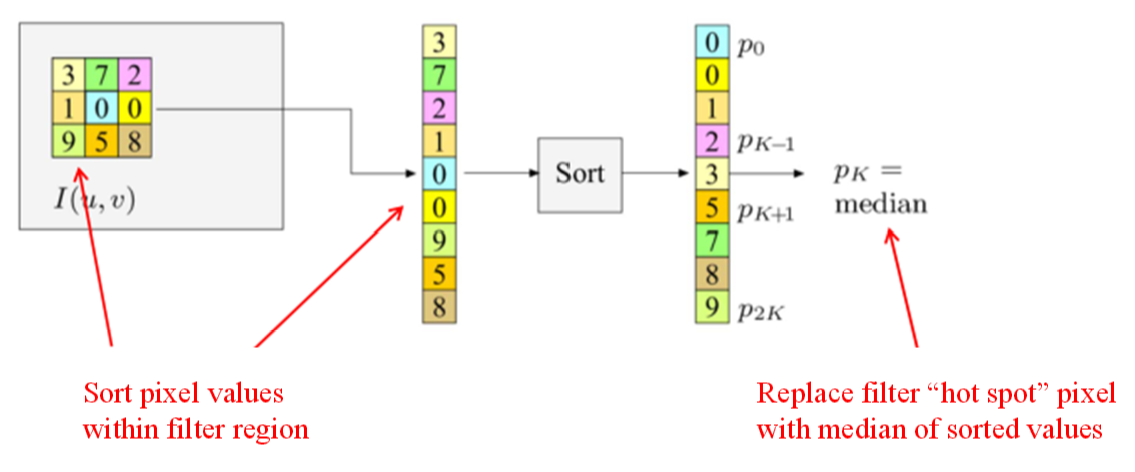如上图示，对于一个3*3大小的滤波器，每次取3*3大小范围内的中值赋值给当前像素。
1.2 中值滤波对图像的影响
如下图示，根据中值滤波器的定义：
（a）单独的一个像素会被消除；（b）单独的一条线会被消除；
（c）阶跃边界保持不变；（d）拐角会被磨圆。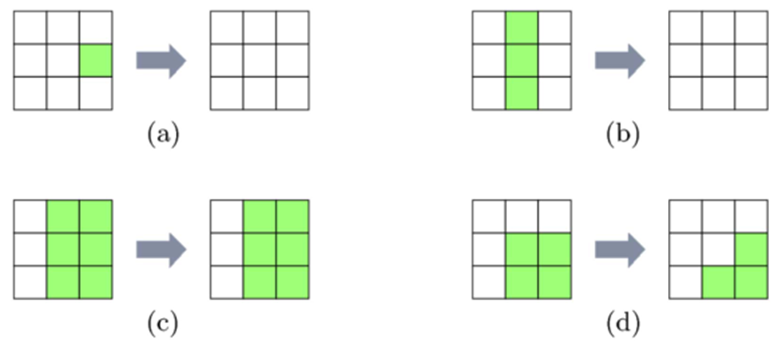因此中值滤波可以很好地去除椒盐噪声，稍后讲到。
1.3 函数编写
function imgMedian = medianfilter(img,filterSize)
% img: image
% filterSize: if=5, it means 5*5

% Generate new blank image
[h, w, c] = size(img);
padSize = (filterSize-1)/2;
imgMedianT = zeros([h+2*padSize, w+2*padSize, c]);
imgMedianT(1+padSize:padSize+h, 1+padSize:padSize+w, :) = img;
imgMedian = zeros([h, w, c]);

for k = 1:c
for i = 1:h
for j = 1:w
block = imgMedianT(i:i-1+filterSize, j:j-1+filterSize, k);
imgMedian(i, j, k) = median(block, 'all');
end
end
end

imgMedian = uint8(imgMedian);

end


1.4 主文件
% imgOrg = rgb2gray(imread("peppers.png"));
imgOrg = imread("peppers.png");
imgOrg = imnoise(imgOrg, 'salt & pepper'); % Adding salt-pepper noise
subplot(1,3,1);
imshow(imgOrg);
title("Originall img");

% median filter
imgMedian = medianfilter(imgOrg, 3); % Generally, the size of a filter is an odd num
subplot(1,3,2);
imshow(imgMedian);
title("Median filter img");

% Using Matlab API
subplot(1,3,3);
[~, ~, c] = size(imgOrg);
newImg = zeros(size(imgOrg));
for i = 1:c
newImg(:,:,i) = medfilt2(imgOrg(:,:,i));
end
newImg = uint8(newImg);
imshow(newImg);
title("Medfilt img by API");

1.5 效果展示可以看到，椒盐噪声被很好地去除了~
2 高效的中值滤波算法
2.1 原理
在每个像素位置上都要对滤波器范围内的所有像素进行排序，这样非常耗时费力。优化[Huang et al., 1979; Pitas and Venetsanopoulos, 1990]后的方法为：当窗口沿行移动一列时，窗口内容的变化只是用右边的一个新列代替了左边的一列，对于m*n的滤波器，（mn-2m）个像素没有变化。
算法步骤：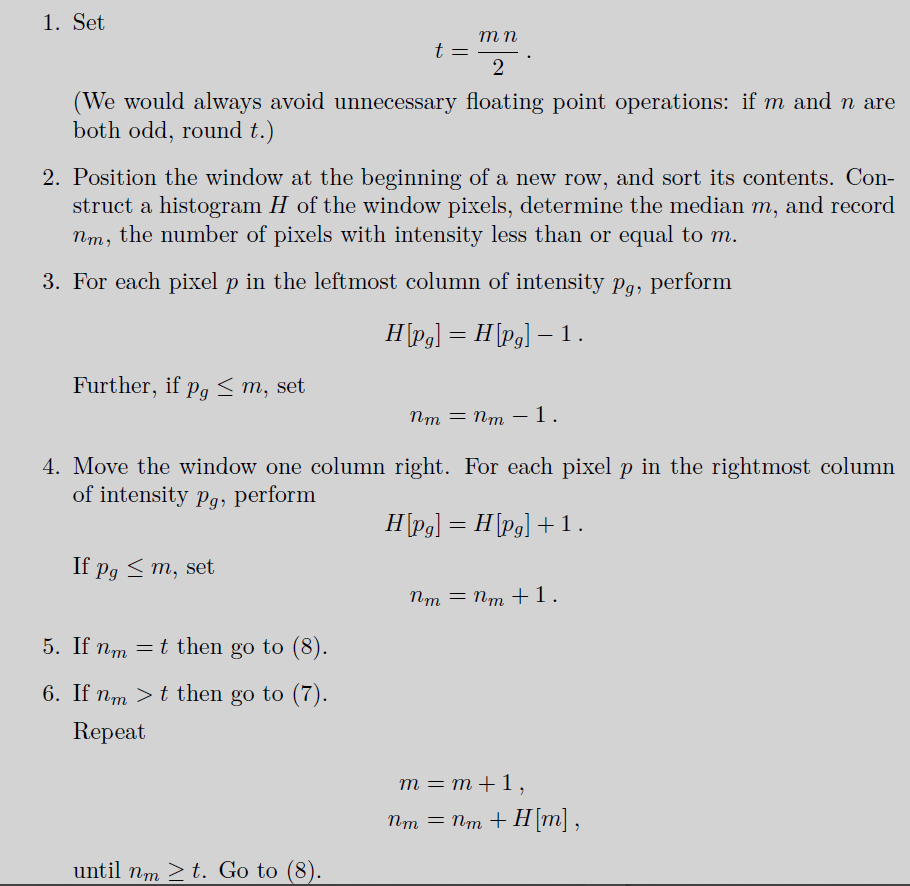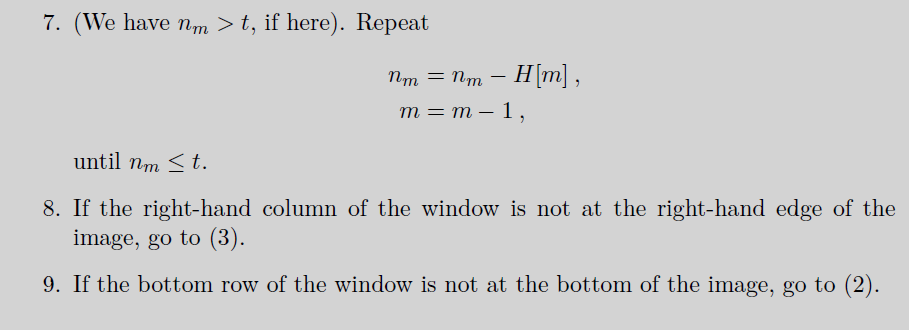2.2 函数编写
请移步Matlab实现图像滤波（叁）：高效的中值滤波查看哦~
3 带权重的中值滤波
3.1 原理
滤波器的每个数字代表了对应位置像素的权重，如下图示，$I(i,j)位置重复W(i,j)次$再进行排序。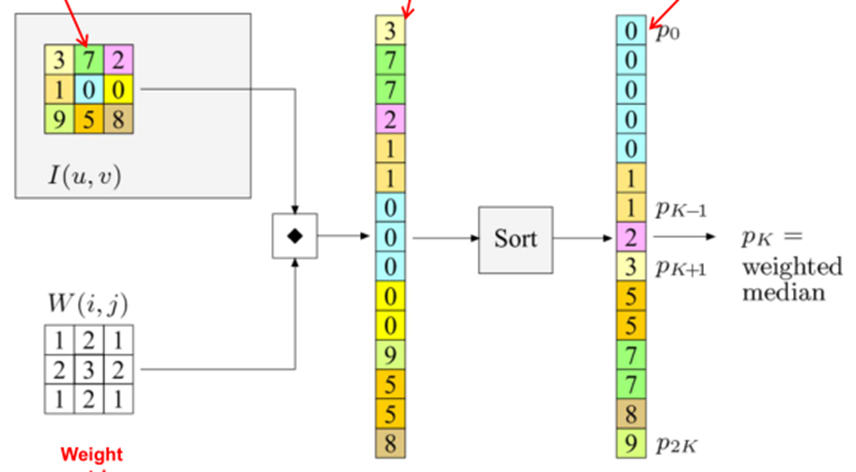3.2 函数编写
function imgwMedianf = wMedianf(img,filter)
% img: image
% filterSize: if=5, it means 5*5

% Generate new blank image
[h, w, c] = size(img);
[hf, wf] = size(filter);
hpadSize = (hf-1)/2;
wpadSize = (wf-1)/2;
imgMedianT = zeros([h+2*hpadSize, w+2*wpadSize, c]);
imgMedianT(1+hpadSize:hpadSize+h, 1+wpadSize:wpadSize+w, :) = img;
imgwMedianf = zeros([h, w, c]);
filter = filter(:);

for k = 1:c
for i = 1:h
for j = 1:w
block = imgMedianT(i:i-1+hf, j:j-1+wf, k);
block = block(:);
block = repelem(block, filter);
imgwMedianf(i, j, k) = median(block, 'all');
end
end
end

imgwMedianf = uint8(imgwMedianf);

end


3.3 主文件
% imgOrg = rgb2gray(imread("peppers.png"));
imgOrg = imread("peppers.png");
imgOrg = imnoise(imgOrg, 'salt & pepper'); % Adding salt-pepper noise
subplot(1,2,1);
imshow(imgOrg);
title("Originall img");

% Weighted Median filter
filter = [1, 2, 1;
2, 3, 2;
1, 2, 1];
imgwMedianf = wMedianf(imgOrg, filter); % Generally, the size of a filter is an odd num
subplot(1,2,2);
imshow(imgwMedianf);
title("Weighted Median filter img");


3.4 效果展示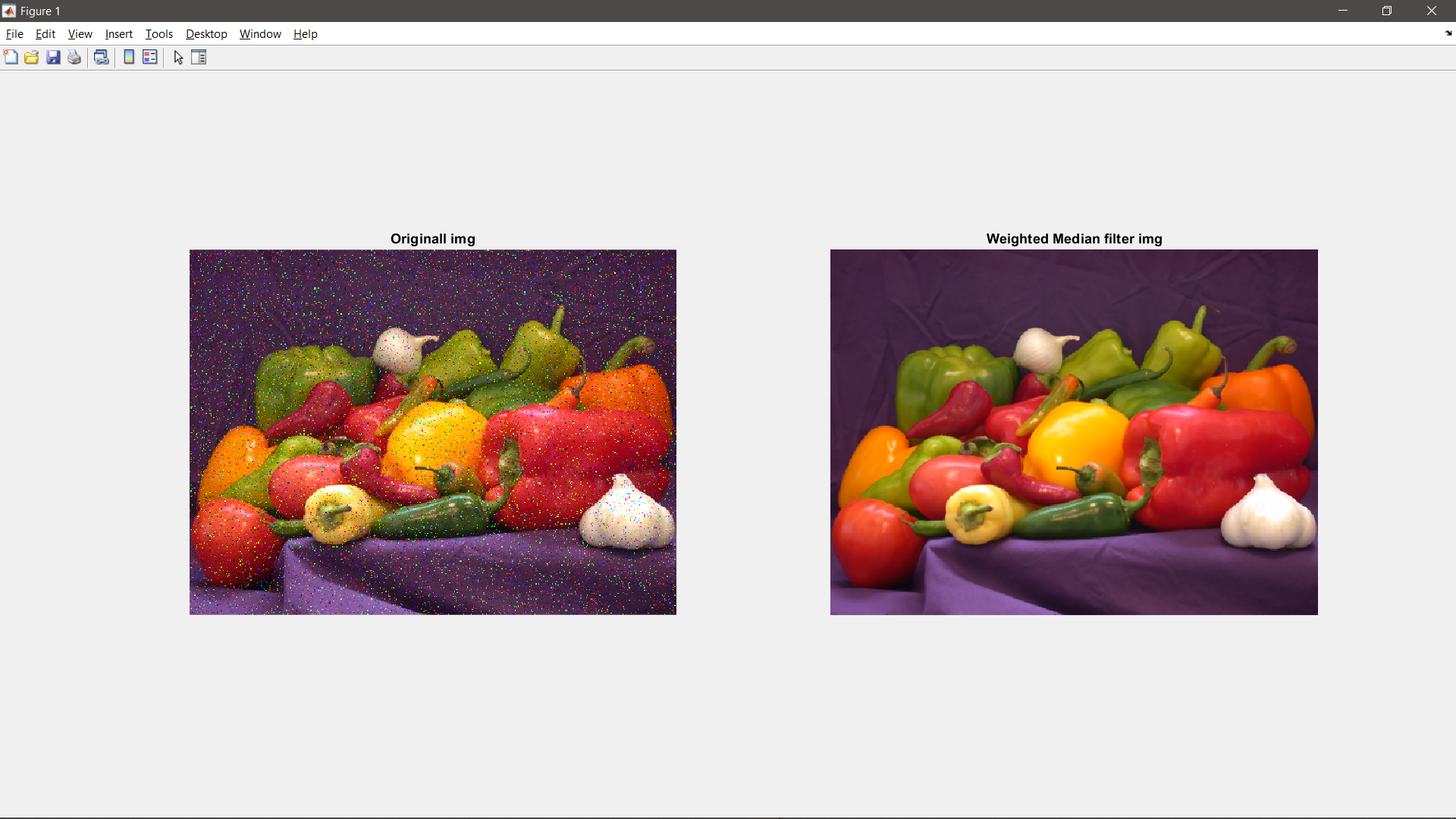展开全文• %中值滤波函数，X为输入矩阵,a,b为中值滤波的框大小。 %效率很低，可以用来说明中值滤波的概念。代码
• Matlab 最大值滤波 中值滤波 最小值滤波 函数 ordfilt2 ...在MATLAB图像处理工具箱中提供了二维统计顺序滤波函数ordfilt2函数。二维统计顺序滤波是中值滤波的推广，对于给定的n个数值｛al ,a2,...,

Matlab 最大值滤波 中值滤波 最小值滤波 函数 ordfilt2

【转载】原文出处：http://blog.csdn.net/fengbingchun/article/details/5951629

ordfilt2函数在MATLAB图像处理工具箱中提供了二维统计顺序滤波函数ordfilt2函数。二维统计顺序滤波是中值滤波的推广，对于给定的n个数值｛al
,a2,...,an｝，将它们按大小顺序排列，将处于第k个位置的元素作为图像滤波输出，即序号为k的二维统计滤波。ordfilt2函数语法格式为：Y=ordfilt2(X,order,domain)
Y=ordfilt2(X,order,domain,S)其功能是：对图像X作顺序统计滤波，order为滤波器输出的顺序值，domain为滤波窗口。S是与domain大小相同的矩阵，它是对应domain中非零值位置的输出偏置，这在图形形态学中是很有用的。例如：Y=ordfilt2(X,5,ones(3,3))，相当于3×3的中值滤波Y=ordfilt2(X,1,ones(3,3))，相当于3×3的最小值滤波Y=ordfilt2(X,9,ones(3,3))，相当于3×3的最大值滤波Y=ordfilt2(X,1,[0 1 0;1 0 1;0 1 0])，输出的是每个像素的东、西、南、北四个方向相邻像素灰度的最小值。

个人备注：

这里，因为模板是3*3，那么邻域内的数据就按1-9由小到大排序，所以函数的第二个参数：5、1、9就分别对应的是中值、最小值、最大值，所以这种参数的设置也就是中值滤波、最小值滤波、最大值滤波。第二个参数的作用取决于模板的大小。

展开全文• MATLAB常用的滤波函数比较：均值滤波和中值滤波 均值滤波中值滤波运行结果程序代码 均值滤波 均值滤波也称为线性滤波，其采用的主要方法为邻域平均法。是典型的线性滤波算法，它是指在图像上对目标像素给一个模板，...图像处理
• Matlab中值滤波去噪（即OpenCv中Blur函数作用替代） 在研究分离聚散焦图片时，用梯度图分离图片后，再加上中值滤波去噪可以达到很好的效果，matlab 均值滤波函数avefilt //均值滤波函数，x是需要滤波的图像，n是模板...opencv 算法
• MATLAB 自适应中值滤波函数adpmedian 参考 冈萨雷斯 《数字图像处理》MATLAB版 function f = adpmedian(g,Smax) if (Smax<=1)||(Smax/2==round(Smax/2))||(Smax~=round(Smax)) error('Smax must be an odd...
• 从运算算子中，选择中间值作为当前输出...中值滤波函数 function [ img ] = ImageMidFilter(imin,operator) [m,n,g] = size(imin); [k,h] = size(operator); imin = im2double(imin); a = fix(k/2); b = f图像处理
• ## Matlab中值滤波去噪

千次阅读 2018-05-10 22:42:44
以灰度图像eight.tif为例，向原始图像中加入椒盐噪声，再对噪声图像调用中值滤波函数midfilt进行去噪。 I=imread('eight.tif'); J=imnoise(I,'salt &amp; pepper'); after=midfilt(J,3); subplot(1,3,1); ...
• Matlab常见的一维数据滤波函数 movmean：平均值滤波 movmedian：中值滤波 此外，同类函数还有获取规定窗口下的标准差与方差函数 movstd：求标准差 ; movvar：求方差 ; 其函数的记忆方法为mov+相应函数的名称 ...
• 实现了数字图像处理中的中值滤波，包含线、十字、X，方形、棱形、圆形窗口的滤波窗口。全都是自己写的，有注释。适用于交作业和研究学习中值滤波的朋友
• 中值滤波取卷积核当中所覆盖像素中的中值作为锚点的像素值即可。此时实验中所用到的为3*3的卷积模板。即3行3列共9个元素的中值作为当前元素的像素值。 二、源代码 close all;clear all;clc; I=imread('image3
• B = HMF(A,N) 使用 NxN 框对矩阵 A 执行混合中值滤波。 混合中值过滤比基于 NxN 平方核的中值过滤器更好地保留边缘，因为来自不同空间方向的数据是分开排列的。 在 NxN 框中计算三个中值：MR 是水平和垂直 R 像素的...
• matlab邻域中值滤波，里面有邻域中值滤波的两种情况处理方式，不超过边界和超过边界用0填充处理，未用matlab中可以直接调用的滤波函数，自己编写的中值滤波函数
• 调用matlab自带函数，对心电信号ECG实现中值滤波，达到去除基线漂移的目的。建议ECG数据长度不要太大，中值滤波运算速度较慢。
• 转自：http://blog.csdn.net/yanqingan/article/details/5738026MatLab自编的均值滤波、中值滤波、高斯滤波 图像处理函数。%自编的均值滤波函数。x是需要滤波的图像,n是模板大小(即n×n)function d=avefiltinput function filter
• ## Matlab中值滤波代码

千次阅读 2016-10-30 19:38:58
img=imread('lena.png'); img=rgb2gray(img) img=im2double(img)%输入图像类型为uint8,将其转换为double类型进行运算 gau=imnoise(img,'gaussian');...%imshow()函数在显示图像时会自动将类型转换为uin
• Matlab 最大值滤波 中值滤波 最小值滤波 函数 ordfilt2 ...在MATLAB图像处理工具箱中提供了二维统计顺序滤波函数ordfilt2函数。二维统计顺序滤波是中值滤波的推广，对于给定的n个数值｛al ,a2,...,ordfilt2
• matlab中值滤波 matlab自带函数： %%matlab中自带的函数 clc clear I=im2double(imread('17.jpg')); figure;imshow(I);title("原图"); [height,width,channel]=size(I); I1=imnoise(I,'salt & pepper'); ...
• 文件中包含test.m文件和两个函数以及一张测试图片。利用学习机会自己编写了均值滤波和中值滤波函数，并在test.m文件中运行，有详细代码注释，希望一起学习。
• 中值滤波的原理、应用及medfilt2函数功能的实现图像处理 矩阵
• MatLab 自编的 均值滤波、中值滤波、高斯滤波 图像处理函数...

# matlab中值滤波函数matlab 订阅